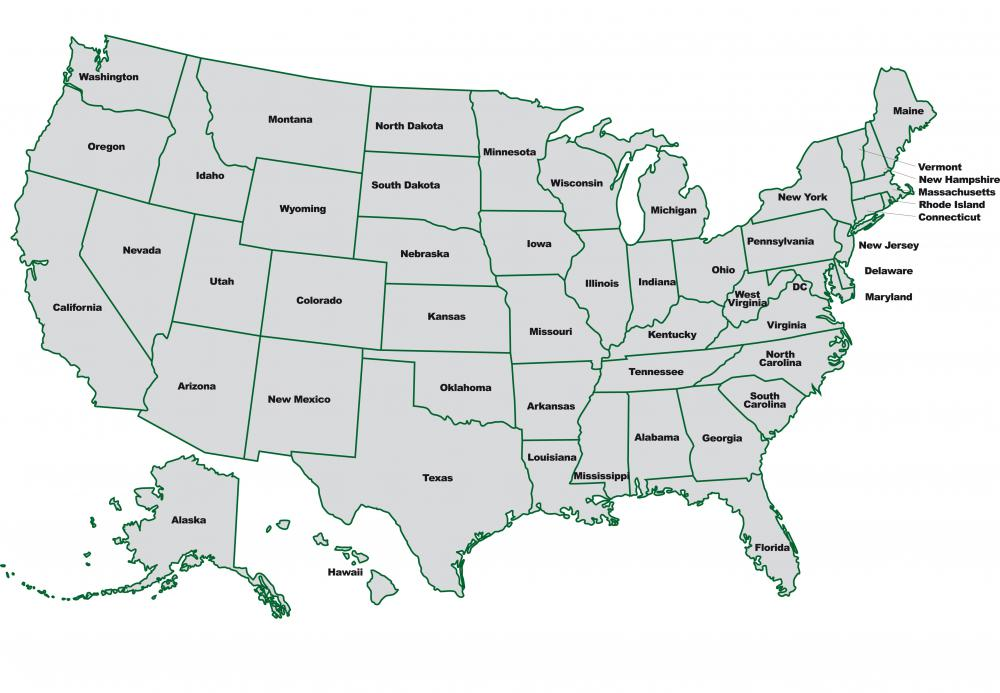# What Is a Hectometer?

Christian Petersen
Christian PetersenIn the United States, the acre remains more common as a unit of measure than the hectare.

A hectometer (hm), or hectometre, as it is usually spelled in countries other than the United States, is a unit of linear measurement that is part of the International System of Units (SI), which is derived from the original metric system. The hectometer is equal to 100 meters and is rarely used as a unit, though derivatives of it are sometimes used, particularly the hectare, which is a unit of area. The cubic hectometer is sometimes used as a unit of volumetric measurement, particularly when discussing large amounts of standing or flowing water, such as reservoirs or rivers.

The term "hectometer," like most of the units in the SI system, is a compound word formed from a base, in this case "meter," and a prefix, "hecto." The prefix "hecto" is used to denote 102, meaning 100. So a hectometer is 102 meters or 100 meters. In modern times, this unit has fallen out of common use, as the meter itself is more often used for smaller distance measurements, and the kilometer is generally used for larger distances. A hectometer, is one tenth of a kilometer and is more likely to be called exactly that rather than a hectometer.

For measurements of area, however, the hectometer has a derived unit, the hectare, that is still in common use. A hectare is one square hectometer, or a square that is 100 meters long on a side. This unit of area is often employed when discussing the size of various divisions of geography, such as the surface area of a body of water, or the size of a field or other piece of land. Even in the United States, which still relies heavily on the old standard or English system, hectares are sometimes used to describe land or open water area, although the acre is still more common as a unit.

The cubic hectometer (hm3 is sometimes used as a unit of volume, usually when describing large amounts of liquids, such as the total volume of a lake or other body of water. It is also sometimes used to describe the flow of rivers. A cubic hectometer is a cube, 100 meters on a side, and is equal to one million cubic meters. It is not necessarily restricted to describing liquid volume, however, and may just as correctly be applied to solid volumes as well.

## You might also Like